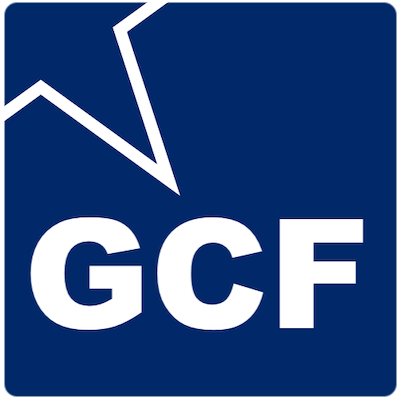Greatest Common Factor AppThe factors of a number are all the integers that you can evenly divide into that number. The Greatest Common Factor (GCF) is the greatest integer that divides exactly into two or more numbers with no remainder.

The Greatest Common Factor is used to simplify fractions. To simplify a fraction, you can divide the numerator and denominator of the fraction by the GCF of the numerator and denominator.

Factors, the Greatest Common Factor, and simplifying fractions go hand-in-hand. Use this app to get the factors of a number, get the Greatest Common Factor of two or more numbers, and to simplify fractions.